# 9.3 Entropy

Learning Objectives

By the end of this section, you will be able to:

• Define entropy
• Explain the relationship between entropy and the number of microstates
• Predict the sign of the entropy change for chemical and physical processes

In 1824, at the age of 28, Nicolas Léonard Sadi Carnot (Figure 9.3.1) published the results of an extensive study regarding the efficiency of steam heat engines. A later review of Carnot’s findings by Rudolf Clausius introduced a new thermodynamic property that relates the spontaneous heat flow accompanying a process to the temperature at which the process takes place. This new property was expressed as the ratio of the reversible heat (qrev) and the kelvin temperature (T). In thermodynamics, a reversible process is one that takes place at such a slow rate that it is always at equilibrium and its direction can be changed (it can be “reversed”) by an infinitesimally small change in some condition. Note that the idea of a reversible process is a formalism required to support the development of various thermodynamic concepts; no real processes are truly reversible, rather they are classified as irreversible.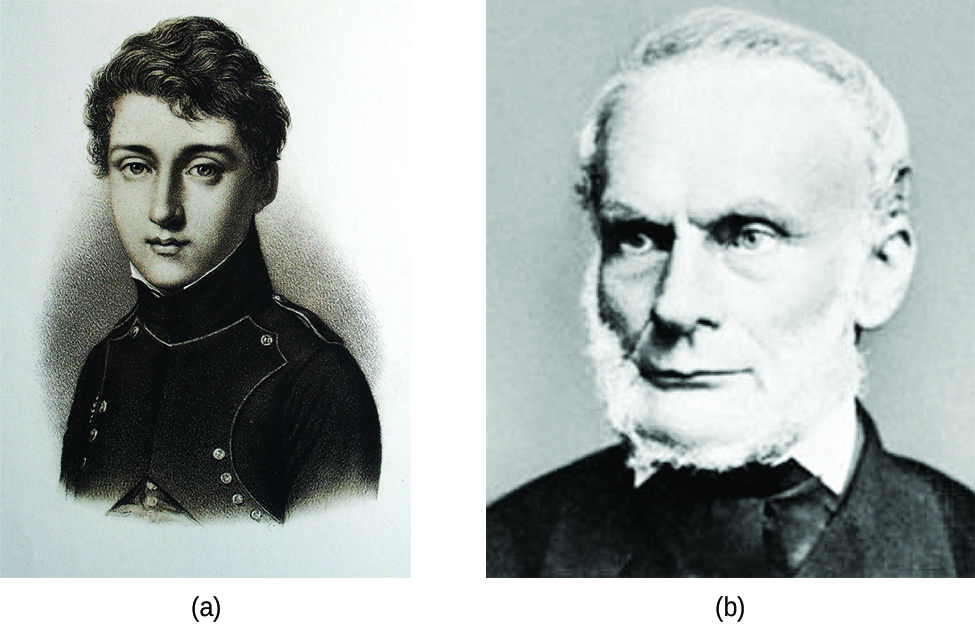Figure 9.3.1 – (a) Nicholas Léonard Sadi Carnot’s research into steam-powered machinery and (b) Rudolf Clausius’s later study of those findings led to groundbreaking discoveries about spontaneous heat flow processes.

Similar to other thermodynamic properties, this new quantity is a state function, so its change depends only upon the initial and final states of a system. In 1865, Clausius named this property entropy (S) and defined its change for any process as the following: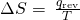The entropy change for a real, irreversible process is then equal to that for the theoretical reversible process that involves the same initial and final states.

## Entropy and Microstates

Following the work of Carnot and Clausius, Ludwig Boltzmann developed a molecular-scale statistical model that related the entropy of a system to the number of microstates (W) possible for the system. A microstate is a specific configuration of all the locations and energies of the atoms or molecules that make up a system. The relation between a system’s entropy and the number of possible microstates is: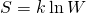where k is the Boltzmann constant, 1.38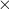10−23 J/K.

As for other state functions, the change in entropy for a process is the difference between its final (Sf) and initial (Si) values: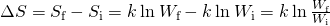For processes involving an increase in the number of microstates, Wf > Wi, the entropy of the system increases and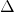S > 0. Conversely, processes that reduce the number of microstates, Wf < Wi, yield a decrease in system entropy,S < 0. This molecular-scale interpretation of entropy provides a link to the probability that a process will occur as illustrated in the next paragraphs.

Consider the general case of a system comprised of N particles distributed among n boxes. The number of microstates possible for such a system is nN. For example, distributing four particles among two boxes will result in 24 = 16 different microstates as illustrated in (Figure 9.3.2). Microstates with equivalent particle arrangements (not considering individual particle identities) are grouped together and are called distributions. The probability that a system will exist with its components in a given distribution is proportional to the number of microstates within the distribution. Since entropy increases logarithmically with the number of microstates, the most probable distribution is therefore the one of greatest entropy.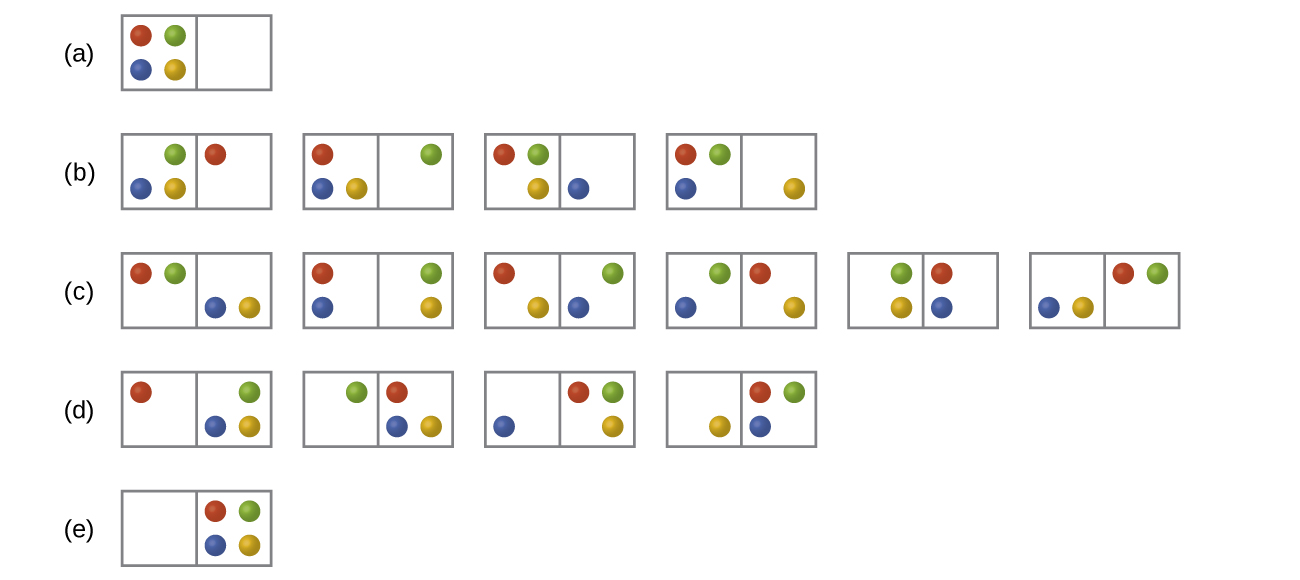Figure 9.3.2 – The sixteen microstates associated with placing four particles in two boxes are shown. The microstates are collected into five distributions—(a), (b), (c), (d), and (e)—based on the numbers of particles in each box.

For this system, the most probable configuration is one of the six microstates associated with distribution (c) where the particles are evenly distributed between the boxes, that is, a configuration of two particles in each box. The probability of finding the system in this configuration is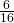or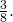The least probable configuration of the system is one in which all four particles are in one box, corresponding to distributions (a) and (e), each with a probability of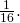The probability of finding all particles in only one box (either the left box or right box) is then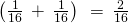or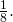As you add more particles to the system, the number of possible microstates increases exponentially (2N). A macroscopic (laboratory-sized) system would typically consist of moles of particles (N ~ 1023), and the corresponding number of microstates would be staggeringly huge. Regardless of the number of particles in the system, however, the distributions in which roughly equal numbers of particles are found in each box are always the most probable configurations.

This matter dispersal model of entropy is often described qualitatively in terms of the disorder of the system. By this description, microstates in which all the particles are in a single box are the most ordered, thus possessing the least entropy. Microstates in which the particles are more evenly distributed among the boxes are more disordered, possessing greater entropy.

The previous description of an ideal gas expanding into a vacuum is a macroscopic example of this particle-in-a-box model. For this system, the most probable distribution is confirmed to be the one in which the matter is most uniformly dispersed or distributed between the two flasks. Initially, the gas molecules are confined to just one of the two flasks. Opening the valve between the flasks increases the volume available to the gas molecules and, correspondingly, the number of microstates possible for the system. Since Wf > Wi, the expansion process involves an increase in entropy (S > 0) and is spontaneous.

A similar approach may be used to describe the spontaneous flow of heat. Consider a system consisting of two objects, each containing two particles, and two units of thermal energy (represented as “*”) in (Figure 9.3.3). The hot object is comprised of particles A and B and initially contains both energy units. The cold object is comprised of particles C and D, which initially has no energy units. Distribution (a) shows the three microstates possible for the initial state of the system, with both units of energy contained within the hot object. If one of the two energy units is transferred, the result is distribution (b) consisting of four microstates. If both energy units are transferred, the result is distribution (c) consisting of three microstates. Thus, we may describe this system by a total of ten microstates. The probability that the heat does not flow when the two objects are brought into contact, that is, that the system remains in distribution (a), is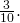More likely is the flow of heat to yield one of the other two distribution, the combined probability being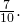The most likely result is the flow of heat to yield the uniform dispersal of energy represented by distribution (b), the probability of this configuration being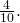This supports the common observation that placing hot and cold objects in contact results in spontaneous heat flow that ultimately equalizes the objects’ temperatures. And, again, this spontaneous process is also characterized by an increase in system entropy.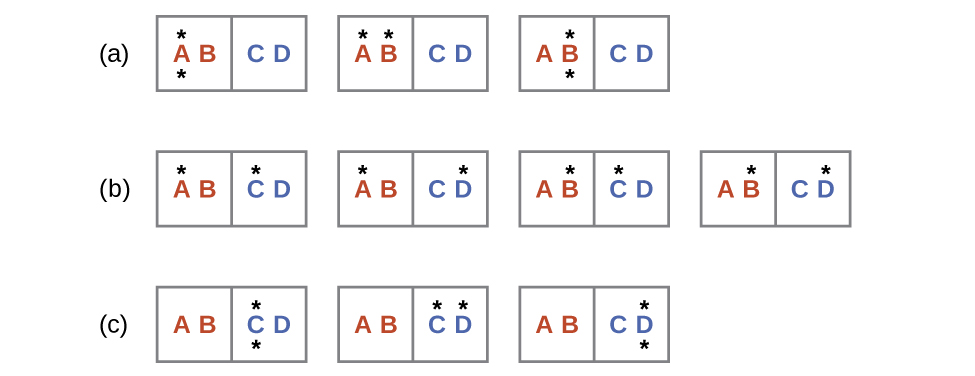Figure 9.3.3 – This shows a microstate model describing the flow of heat from a hot object to a cold object. (a) Before the heat flow occurs, the object comprised of particles A and B contains both units of energy and as represented by a distribution of three microstates. (b) If the heat flow results in an even dispersal of energy (one energy unit transferred), a distribution of four microstates results. (c) If both energy units are transferred, the resulting distribution has three microstates.

Activity 9.3.1 – Determination ofS

Calculate the change in entropy for the process depicted below.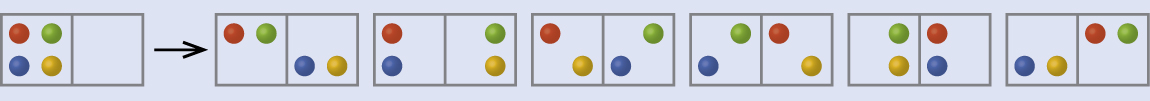## Solution

The initial number of microstates is one, the final six: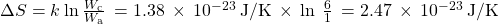The sign of this result is consistent with expectation; since there are more microstates possible for the final state than for the initial state, the change in entropy should be positive.

Consider the system shown in (Figure 9.3.3). What is the change in entropy for the process where all the energy is transferred from the hot object (AB) to the cold object (CD)?

0 J/K

## Predicting the Sign ofS

The relationships between entropy, microstates, and matter/energy dispersal described previously allow us to make generalizations regarding the relative entropies of substances and to predict the sign of entropy changes for chemical and physical processes. Consider the phase changes illustrated in (Figure 9.3.4). In the solid phase, the atoms or molecules are restricted to nearly fixed positions with respect to each other and are capable of only modest oscillations about these positions. With essentially fixed locations for the system’s component particles, the number of microstates is relatively small. In the liquid phase, the atoms or molecules are free to move over and around each other, though they remain in relatively close proximity to one another. This increased freedom of motion results in a greater variation in possible particle locations, so the number of microstates is correspondingly greater than for the solid. As a result, Sliquid > Ssolid and the process of converting a substance from solid to liquid (melting) is characterized by an increase in entropy,S > 0. By the same logic, the reciprocal process (freezing) exhibits a decrease in entropy,S < 0.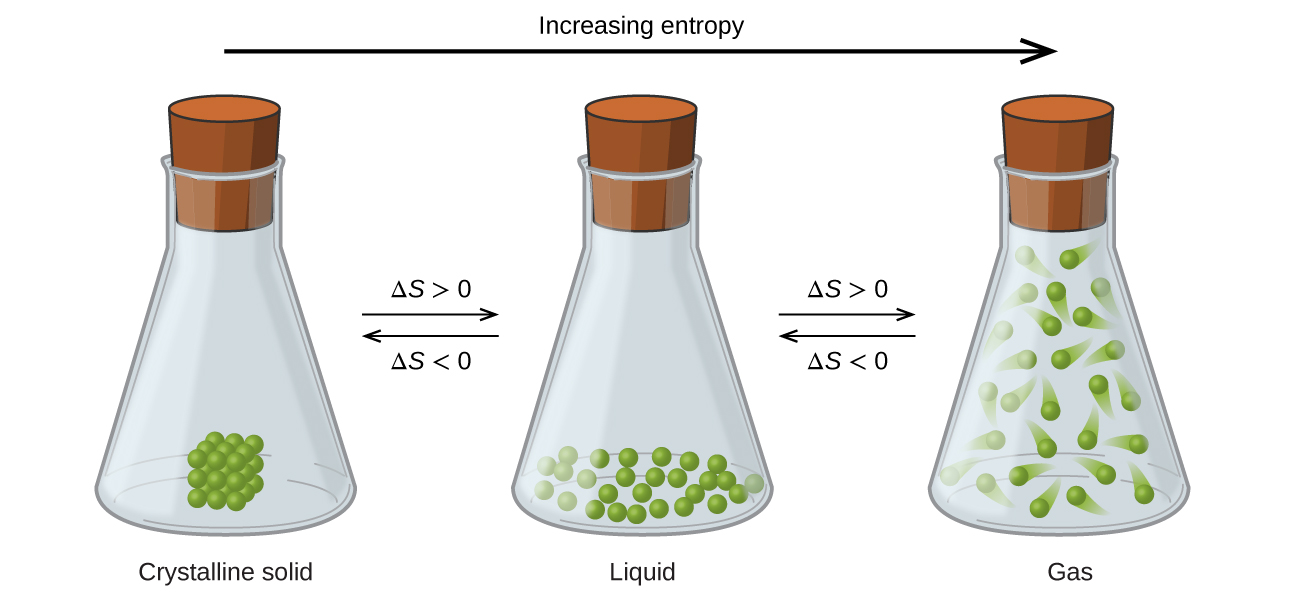Figure 9.3.4 – The entropy of a substance increases (S > 0) as it transforms from a relatively ordered solid, to a less-ordered liquid, and then to a still less-ordered gas. The entropy decreases (S < 0) as the substance transforms from a gas to a liquid and then to a solid.

Now consider the gaseous phase, in which a given number of atoms or molecules occupy a much greater volume than in the liquid phase. Each atom or molecule can be found in many more locations, corresponding to a much greater number of microstates. Consequently, for any substance, Sgas > Sliquid > Ssolid, and the processes of vaporization and sublimation likewise involve increases in entropy,S > 0. Likewise, the reciprocal phase transitions, condensation and deposition, involve decreases in entropy,S < 0.

According to kinetic-molecular theory, the temperature of a substance is proportional to the average kinetic energy of its particles. Raising the temperature of a substance will result in more extensive vibrations of the particles in solids and more rapid translations of the particles in liquids and gases. At higher temperatures, the distribution of kinetic energies among the atoms or molecules of the substance is also broader (more dispersed) than at lower temperatures. Thus, the entropy for any substance increases with temperature (Figure 9.3.5).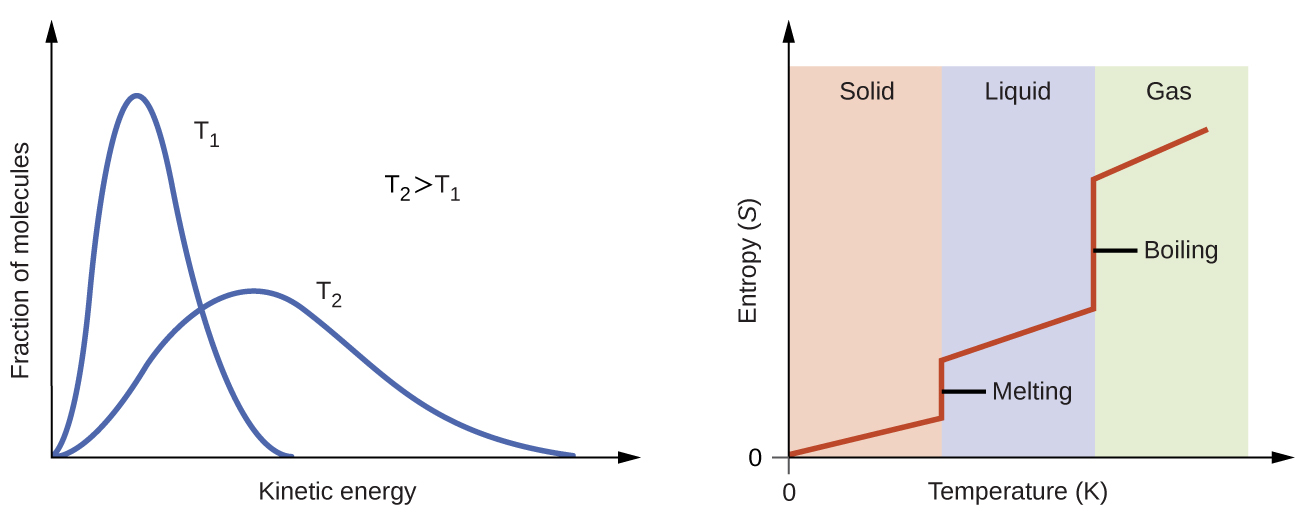Figure 9.3.5 – Entropy increases as the temperature of a substance is raised, which corresponds to the greater spread of kinetic energies. When a substance undergoes a phase transition, its entropy changes significantly.

The entropy of a substance is influenced by the structure of the particles (atoms or molecules) that comprise the substance. With regard to atomic substances, heavier atoms possess greater entropy at a given temperature than lighter atoms, which is a consequence of the relation between a particle’s mass and the spacing of quantized translational energy levels (a topic beyond the scope of this text). For molecules, greater numbers of atoms increase the number of ways in which the molecules can vibrate and thus the number of possible microstates and the entropy of the system.

Finally, variations in the types of particles affects the entropy of a system. Compared to a pure substance, in which all particles are identical, the entropy of a mixture of two or more different particle types is greater. This is because of the additional orientations and interactions that are possible in a system comprised of nonidentical components. For example, when a solid dissolves in a liquid, the particles of the solid experience both a greater freedom of motion and additional interactions with the solvent particles. This corresponds to a more uniform dispersal of matter and energy and a greater number of microstates. The process of dissolution therefore involves an increase in entropy,S > 0.

Considering the various factors that affect entropy allows us to make informed predictions of the sign ofS for various chemical and physical processes.

Activity 9.3.2 – Predicting the Sign of ∆S

Predict the sign of the entropy change for the following processes. Indicate the reason for each of your predictions.

(a) One mole liquid water at room temperature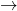one mole liquid water at 50 °C

(b)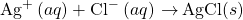(c)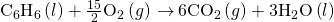(d)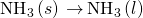## Solution

(a) positive, temperature increases

(b) negative, reduction in the number of ions (particles) in solution, decreased dispersal of matter

(c) negative, net decrease in the amount of gaseous species

(d) positive, phase transition from solid to liquid, net increase in dispersal of matter

Predict the sign of the entropy change for the following processes. Give a reason for your prediction.

(a)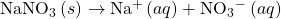(b) the freezing of liquid water

(c)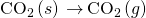(d)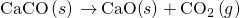(a) Positive; The solid dissolves to give an increase of mobile ions in solution. (b) Negative; The liquid becomes a more ordered solid. (c) Positive; The relatively ordered solid becomes a gas. (d) Positive; There is a net increase in the amount of gaseous species.

# Key Concepts and Summary

Entropy (S) is a state function that can be related to the number of microstates for a system (the number of ways the system can be arranged) and to the ratio of reversible heat to kelvin temperature. It may be interpreted as a measure of the dispersal or distribution of matter and/or energy in a system, and it is often described as representing the “disorder” of the system.

For a given substance, entropy depends on phase with Ssolid < Sliquid < Sgas. For different substances in the same physical state at a given temperature, entropy is typically greater for heavier atoms or more complex molecules. Entropy increases when a system is heated and when solutions form. Using these guidelines, the sign of entropy changes for some chemical reactions and physical changes may be reliably predicted.

## Key Equations

•• S = k ln W
•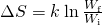End of Chapter Exercises

(1) In (Figure 9.3.2) all possible distributions and microstates are shown for four different particles shared between two boxes. Determine the entropy change,S, if the particles are initially evenly distributed between the two boxes, but upon redistribution all end up in Box (b).

(2) In (Figure 9.3.2) all of the possible distributions and microstates are shown for four different particles shared between two boxes. Determine the entropy change,S, for the system when it is converted from distribution (b) to distribution (d).

Solution

There are four initial microstates and four final microstates.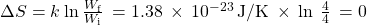(3) Consider a system similar to the one in (Figure 9.3.2), except that it contains six particles instead of four. What is the probability of having all the particles in only one of the two boxes in the case? Compare this with the similar probability for the system of four particles that we have derived to be equal toWhat does this comparison tell us about even larger systems?

Solution

The probability for all the particles to be on one side is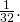This probability is noticeably lower than theresult for the four-particle system. The conclusion we can make is that the probability for all the particles to stay in only one part of the system will decrease rapidly as the number of particles increases, and, for instance, the probability for all molecules of gas to gather in only one side of a room at room temperature and pressure is negligible since the number of gas molecules in the room is very large.

(4) Consider the system shown in (Figure 9.3.3). What is the change in entropy for the process where the energy is initially associated only with particle A, but in the final state the energy is distributed between two different particles?

(5) Consider the system shown in (Figure 9.3.3). What is the change in entropy for the process where the energy is initially associated with particles A and B, and the energy is distributed between two particles in different boxes (one in A-B, the other in C-D)?

Solution

There is only one initial state. For the final state, the energy can be contained in pairs A-C, A-D, B-C, or B-D. Thus, there are four final possible states.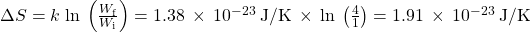(6) Arrange the following sets of systems in order of increasing entropy. Assume one mole of each substance and the same temperature for each member of a set.

(6a) H2(g), HBrO4(g), HBr(g)

(6b) H2O(l), H2O(g), H2O(s)

(6c) He(g), Cl2(g), P4(g)

(7) At room temperature, the entropy of the halogens increases from I2 to Br2 to Cl2. Explain.

Solution

The masses of these molecules would suggest the opposite trend in their entropies. The observed trend is a result of the more significant variation of entropy with a physical state. At room temperature, I2 is a solid, Br2 is a liquid, and Cl2 is a gas.

(8) Consider two processes: sublimation of I2(s) and melting of I2(s) (Note: the latter process can occur at the same temperature but somewhat higher pressure).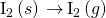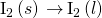IsS positive or negative in these processes? In which of the processes will the magnitude of the entropy change be greater?

(9) Indicate which substance in the given pairs has the higher entropy value. Explain your choices.

(9a) C2H5OH(l) or C3H7OH(l)

(9b) C2H5OH(l) or C2H5OH(g)

(9c) 2H(g) or H(g)

Solution

(a) C3H7OH(l) as it is a larger molecule (more complex and more massive), and so more microstates describing its motions are available at any given temperature. (b) C2H5OH(g) as it is in the gaseous state. (c) 2H(g), since entropy is an extensive property, and so two H atoms (or two moles of H atoms) possess twice as much entropy as one atom (or one mole of atoms).

(10) Predict the sign of the entropy change for the following processes.

(10a) An ice cube is warmed to near its melting point.

(10b) Exhaled breath forms fog on a cold morning.

(10c) Snow melts.

(11) Predict the sign of the entropy change for the following processes. Give a reason for your prediction.

(11a)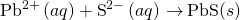(11b)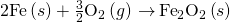(11c)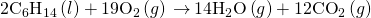Solution

(a) Negative. The relatively ordered solid precipitating decreases the number of mobile ions in solution. (b) Negative. There is a net loss of three moles of gas from reactants to products. (c) Positive. There is a net increase of seven moles of gas from reactants to products.

(12) Write the balanced chemical equation for the combustion of methane, CH4(g), to give carbon dioxide and water vapor. Explain why it is difficult to predict whetherS is positive or negative for this chemical reaction.

(13) Write the balanced chemical equation for the combustion of benzene, C6H6(l), to give carbon dioxide and water vapor. Would you expectS to be positive or negative in this process?

Solution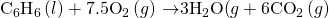There are 7.5 moles of gas initially, and 3 + 6 = 9 moles of gas in the end. Therefore, it is likely that the entropy increases as a result of this reaction, andS is positive.

## Glossary

state function that is a measure of the matter and/or energy dispersal within a system, determined by the number of system microstates; often described as a measure of the disorder of the system
possible configuration or arrangement of matter and energy within a system
process that takes place so slowly as to be capable of reversing direction in response to an infinitesimally small change in conditions; hypothetical construct that can only be approximated by real processes